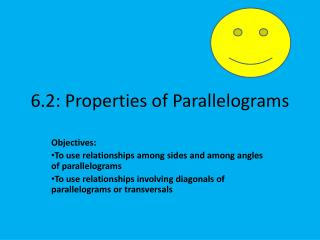DownloadDownload Presentation6.2: Properties of Parallelograms

# 6.2: Properties of Parallelograms

Download Presentation## 6.2: Properties of Parallelograms

- - - - - - - - - - - - - - - - - - - - - - - - - - - E N D - - - - - - - - - - - - - - - - - - - - - - - - - - -
##### Presentation Transcript

1. 6.2: Properties of Parallelograms Objectives: To use relationships among sides and among angles of parallelograms To use relationships involving diagonals of parallelograms or transversals

2. Please note… • Every property that you learn in this section also applies to rectangles, rhombuses and squares!!!!! They are part of the Parallelogram Family! • The converse of every theorem in this section is also true.

3. THEOREM: Opposite sides of a parallelogram ( ) are congruent.

4. Example: Given EFGH Find GH and EG 7 E F 6 G H

5. Theorem: Opposite angles of a are congruent. W V T U

6. EXAMPLE C J 115 65 G H FIND measure of angle J and angle H.

7. Consecutive Angles • Angles that share a side D C A B

8. In a parallelogram, consecutive angles are supplementary (Because they are same-side interior angles!!) D C A B

9. Find the values of the variables. b+7 c+4 2c 60° (3a)° 18

10. Theorem: The diagonals of a bisect each other. E F K H G Example: If EK = 4 and HK = 7, find KG and KF

11. The figure below is a parallelogram. Find the value of x. A 2x-1 AD=26 D

12. The figure below is a parallelogram. Find the values of x and y. 2x+3 6y-16 4y 4x-9

13. Theorem If one pair of opposite sides of a quadrilateral is BOTH congruent and parallel, then the quadrilateral is a parallelogram. EXAMPLES: Is there enough information to determine that the quadrilateral is a parallelogram? Explain. a.) b.) x x

14. Graph the parallelogram. Reflect it over the x –axis. A(1,4), B(3,5) , C(6,1), D(4,0)

15. Theorem: If 3 or more parallel lines cut off congruent segments on one transversal, then they cut off congruent segments on EVERY transversal.